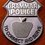# If any one could solve this problem

Where did the one rupee go?

Suppose Tom and Harry have 30 lemons each. Tom sells at the rate of 3 lemons for Rs. 1 and Harry sells at the rate of 2 lemons for Rs. 1. So in the end Tom earns 30 / 3 * 1= 10 Rupees and Harry earns 30 / 2 * 1 = 15 Rupees. So total of 10 + 15 = 25 Rs for 30 + 30 = 60 lemons.

One day Tom leaves out leaving all lemons to Harry. So Harry sells 3 + 2 = 5 lemons for 1 + 1 = 2 rupees. So in the end Harry sells 60/5 * 2 = 12 * 2 = 24 rupees.

So there comes out a difference of 1 rupee. So where did that one rupee go?Note by Praveen Rajput
5 years, 8 months ago

This discussion board is a place to discuss our Daily Challenges and the math and science related to those challenges. Explanations are more than just a solution — they should explain the steps and thinking strategies that you used to obtain the solution. Comments should further the discussion of math and science.

When posting on Brilliant:

• Use the emojis to react to an explanation, whether you're congratulating a job well done , or just really confused .
• Ask specific questions about the challenge or the steps in somebody's explanation. Well-posed questions can add a lot to the discussion, but posting "I don't understand!" doesn't help anyone.
• Try to contribute something new to the discussion, whether it is an extension, generalization or other idea related to the challenge.
• Stay on topic — we're all here to learn more about math and science, not to hear about your favorite get-rich-quick scheme or current world events.

MarkdownAppears as
*italics* or _italics_ italics
**bold** or __bold__ bold
- bulleted- list
• bulleted
• list
1. numbered2. list
1. numbered
2. list
Note: you must add a full line of space before and after lists for them to show up correctly
paragraph 1paragraph 2

paragraph 1

paragraph 2

[example link](https://brilliant.org)example link
> This is a quote
This is a quote
    # I indented these lines
# 4 spaces, and now they show
# up as a code block.

print "hello world"
# I indented these lines
# 4 spaces, and now they show
# up as a code block.

print "hello world"
MathAppears as
Remember to wrap math in $$ ... $$ or $ ... $ to ensure proper formatting.
2 \times 3 $2 \times 3$
2^{34} $2^{34}$
a_{i-1} $a_{i-1}$
\frac{2}{3} $\frac{2}{3}$
\sqrt{2} $\sqrt{2}$
\sum_{i=1}^3 $\sum_{i=1}^3$
\sin \theta $\sin \theta$
\boxed{123} $\boxed{123}$

## Comments

Sort by:

Top Newest

There is a difference between the two ways of selling the lemons:

1. One person sells lemons at the rate of 5 lemon for Rs. 2

2. One person sells lemons at the rate of 3 lemons for Rs.1, then the next 2 lemons for Rs.1, then the next 3 lemons for Rs. 1 and so on.

Suppose there are 8 lemons. If the one sells them at the rate of 5 lemons for Rs. 2. then the total cost you will charge for the lemons is $\frac 25 * 8=3.2$ rupees.

But if you sell them same as the second way. Then you will get $1+1+1=3$ rupees for 8 lemons.

So where did Rs $0.2$ go?

- 5 years, 8 months ago

Log in to reply

Yeah you are right because there are two other way of selling mentioned above which has a really big difference.

- 5 years, 8 months ago

Log in to reply

yes, this problem was asked by my maths teacher to me when I was in the 4th grade and doing maths great, but the question baffled me for years and still has, we are selling the same thing to same cost but is it possible that it gives different answers

- 5 years, 8 months ago

Log in to reply

No rupee is lost. With this pairinrg 2 & 3 for2rs for 30 minimum is 24. It is # of turns of small # in pair the item is sold. One can gain 1-5 rs more by increasing times of smal # in pairing keeping rate constant using following way. A Sell 20 (2 for 1rs):20 & 40(5 for 2):16 Total 26rs. B Sell 20(5for2):8 & 40(2for1)::20 Total 28rs. C 60(2for1):30& 0(5for2):0 Total 30rs. This is used as marketing tacticts for max profit.

- 5 years, 8 months ago

Log in to reply

I think the last step( i.e. after tom leaving all lemons to Harry) is wrong. You need to do the individual calculation because the rates of lemons are different( Rs.1 for 2 lemons and rs.1 for 3 lemons.). You cannot just add up like that.

- 5 years, 8 months ago

Log in to reply

×

Problem Loading...

Note Loading...

Set Loading...